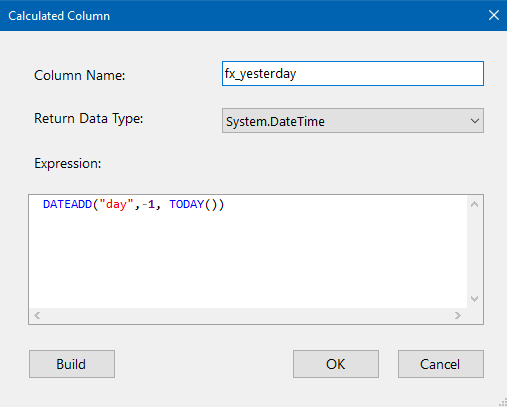Functions

Calculates the addition of an amount of time to a DateTime, this will return the DateTime after the specified amount has been added to it. Can be used to subtract an amount of time to a DateTime.

## Syntax

``````DateTime? DATEADD(string part, int num, DateTime? date)
``````

## Input

Object Data Type Description
part String A string to represent the time unit
• year
• month
• day
• hour
• minute
• second
num Integer An integer representing the amount of time to add.
date DateTime A DateTime value.

## Examples

In this example we will imagine that we want to calculate the expiry date of trial keys which will expire 15 days after the start date, we have a StartDate column for each record available within our dataset.

``````DATEADD("day", 15, StartDate)
``````

If StartDate = 15/09/2017 then the above expression will return '30/09/2017'.

To subtract an amount of time:

Imagine that we want to return everything that was created/modified 7 days before today.

``````DATEADD("day",-7, TODAY())
``````

When you run the above example it will return a DateTime seven days prior to today. We can then use this date with a LookUp on a CreatedDate or ModifiedDate to return all records that were created/modified on that date.

To return yesterday you can use:

``````DATEADD("day",-1, TODAY())
``````